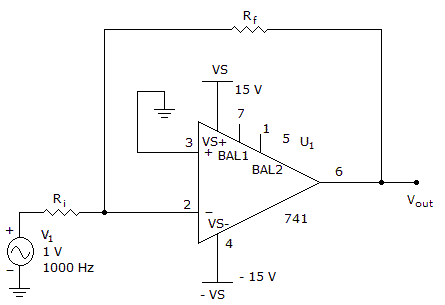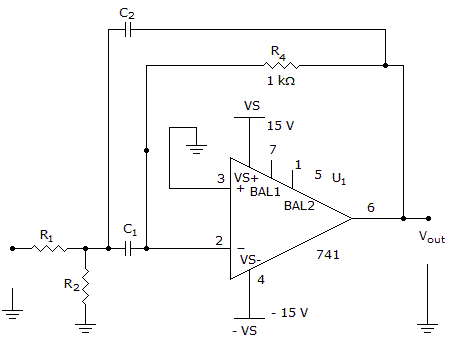# Electronics - Operational Amplifiers

### Exercise :: Operational Amplifiers - True or False

1.

Feedback is used in an open-loop operational amplifier circuit.

 A. True B. False

Explanation:

No answer description available for this question. Let us discuss.

2.

This circuit is operating in a closed-loop mode.A. True B. False

Explanation:

No answer description available for this question. Let us discuss.

3.

This circuit is a bandpass filter.A. True B. False

Explanation:

No answer description available for this question. Let us discuss.

4.

This circuit is a voltage follower.A. True B. False

Explanation:

No answer description available for this question. Let us discuss.

5.

If the two inputs to a differential amplifier are exactly the same, then the output is the signal multiplied by two.

 A. True B. False